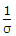# Electronics and Communication Engineering - Electronic Devices and Circuits

1.
An incremental model of a solid state device is one which represents the
ac property of the device at desired operating point
dc property of the device at all operating points
complete ac and dc behaviour at all operating points
ac property of the device at all operating points
Explanation:

Incremental model is used for ac response at one operating point.

2.
What is the correct sequence of the following step in the fabrication of a monolithic, Bipolar junction transistor?
1. Emitter diffusion
2. Base diffusion
3. Buried layer formation
4. E pi-layer formation
Select the correct answer using the codes given below:
3, 4, 1, 2
4, 3, 1, 2
3, 4, 2, 1
4, 3, 2, 1
Explanation:

It is always non-linear.

3.
For an n-channel enhancement type MOSFET, if the source is connected at a higher potential than that of the bulk (VSB > 0), the threshold voltage VT of the MOSFET will
remain unchanged
decrease
change Polarity
increase
Explanation:

VT depends upon MOSFET construction, hence it will Independent from MOSFET parameters.

4.
Which of the following is used for generating time varying wave forms?
MOSFET
PIN diode
Tunnel diode
UJT
Explanation:

Its output is used to trigger SCR.

5.
Calculate the resistivity of n-type semiconductor from the following data, Density of holes = 5 x 1012 cm-3. Density of electrons = 8 x 1013 cm-3, mobility of conduction electron = 2.3 x 104 cm2/ V-sec and mobility of holes = 100 cm2/V-sec.
0.43 Ω-m
0.34 Ω-m
0.42 Ω-m
0.24 Ω-m
Resistivity(r) =.σ = e(neue + nnun).